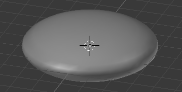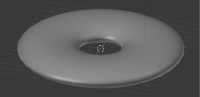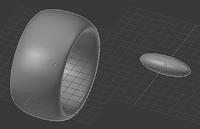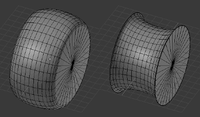# Finding the volume of an ellipse

#### david123

##### New member
I came across this calculus problem that asked me to find the volume of the ellipse (x^2)/9 + y^2 = 1 rotated around the line y = 5. What I don't understand is that before you even attempt the problem you have to shift it down 5 units so that the center is at (0, -5). My question is why do you have to shift it down 5 units. I got confused because in calculus when you rotate a function around a line you don't usually move the function anywhere, it stays in one spot.

#### Subhotosh Khan

##### Super Moderator
Staff member
I came across this calculus problem that asked me to find the volume of the ellipse (x^2)/9 + y^2 = 1 rotated around the line y = 5. What I don't understand is that before you even attempt the problem you have to shift it down 5 units so that the center is at (0, -5). My question is why do you have to shift it down 5 units. I got confused because in calculus when you rotate a function around a line you don't usually move the function anywhere, it stays in one spot.
I suppose you are referring to "hints" provided for solution - or you are looking at the solution.

You are correct. You don't have to shift the ellipse. Have you tried to solve the problem without shifting?

If you do not shift - what answer do you get?

#### HallsofIvy

##### Elite Member
Let (x, y) be a general point inside the ellipse. Rotating that point around the line y= 5, it sweeps out a circle with radius 5- y and so circumference $$\displaystyle 2\pi(5- y)$$. A tiny rectangle of sides dx and dy, so of area dxdy, sweeps out a volume $$\displaystyle 2\pi(5- y)dxdy$$. Integrate that over all x, y in the ellipse.

#### Cubist

##### Full Member
The formula for "volume of revolution" usually considers a revolution around the x or y axis (in my experience). Here is what the ellipse equation looks like when revolved around the y axis...And after shifting...The volumes will be different. The question requires the volume of the second shape.

Don't worry, both of these shapes have been safely stashed away in area 51.

#### Cubist

##### Full Member
BTW: The formula (click). Just search for "volume of revolution" for other pages on this.

NB: HallsofIvy's approach would probably work too.

#### Cubist

##### Full Member
To use the formula for this particular shape you'd have to split the ellipse into two - an outer and inner half. The inner volume would be subtracted from the outer to account for the "empty volume" in the middle of the donut. If you need more detail about this then please ask. But I guess that you're reading about this example in a text book, therefore you might not need more explanation?

#### Cubist

##### Full Member
The formula for "volume of revolution" usually considers a revolution around the x or y axis (in my experience). Here is what the ellipse equation looks like when revolved around the y axis...

View attachment 16608

And after shifting...
View attachment 16609

The volumes will be different. The question requires the volume of the second shape.

Don't worry, both of these shapes have been safely stashed away in area 51.
I just spotted that I rotated the ellipse around x=5, not y=5. Off to the corner with me! (or perhaps to area 51?)

However the pricipal of my posts should still hold even though the shapes will look different. If I get time later I'll post a corrected 3d view.

#### HallsofIvy

##### Elite Member
I've always assumed you were from area 51!

•Cubist

#### Cubist

##### Full Member
See below for rotation around the x axis. The left shape has had the shift applied before rotation...And to calculate the volume of the above (left) shape, take the difference of the following two volumes:- LHS minus RHS.These shapes correspond to the lower and upper half of the ellipse being revolved separately.

#### firemath

##### Full Member
See below for rotation around the x axis. The left shape has had the shift applied before rotation...

View attachment 16613

And to calculate the volume of the above (left) shape, take the difference of the following two volumes:- LHS minus RHS.

View attachment 16614

These shapes correspond to the lower and upper half of the ellipse being revolved separately.
Tires for your alien spaceship?#### Cubist

##### Full Member
Tires for your alien spaceship?and a cigar for when the calculation is complete!

•firemath

#### Subhotosh Khan

##### Super Moderator
Staff member
I came across this calculus problem that asked me to find the volume of the ellipse (x^2)/9 + y^2 = 1 rotated around the line y = 5. What I don't understand is that before you even attempt the problem you have to shift it down 5 units so that the center is at (0, -5). My question is why do you have to shift it down 5 units. I got confused because in calculus when you rotate a function around a line you don't usually move the function anywhere, it stays in one spot.
Approximately sketch the ellipse - the major axis of the ellipse is x-axis.

When you rotate the ellipse about y = 5, the "tire" above will be coming-out and going-in through z-direction. If you take a cross-section of the rotated-volume by the x-y plane - you will get two ellipses on the x-y plane. One with the major axis along y=0 (x-axis) and the other will be x-axis along y = 10. I think the shift you are talking about is at y=10. Then use washer method to calculate volume.

#### Jomo

##### Elite Member
If you are correct that you want the volume of the ellipse, then you can rotate about the origin.
In fact if you were given, for example, $$\displaystyle \dfrac{(x-2)^2}{4} + \dfrac{(y-3)^2}{9}=1$$ and you want the volume you can rotate about y=3 and this will get you the correct result. However you can, if you like, move the ellipse so that its center goes from (2,3) to (0,0) by using the equation $$\displaystyle \dfrac{x^2}{4} + \dfrac{y^2}{9}=1$$ and rotate about y=0. You can do this for any volume problem.

#### Jomo

##### Elite Member
I got confused because in calculus when you rotate a function around a line you don't usually move the function anywhere, it stays in one spot.
Here is the difference. When you rotate a function (a 3-d figure) about y=5 you are not (necessarily) finding the volume of the given figure, as the volume seen in the rotation is not the same as the original figure. Picture a cylinder, where one side is along y=2, and the other side is along y=0. x goes from 0 to 5. Now if you rotate this figure along y=1 you will find the volume of the cylinder. PLEASE draw this and think about it until you are positive that I am correct. Now consider rotating about y=5. Do you see that you are getting a different volume (not numerically different but a different looking object). When you integrate this, you are finding the volume of this new figure, not the original cylinder.

Now back to you original problem. Since you want the volume of the ellipse, NOT the volume of what you get when you revolve the ellipse about y=5, you can NOT revolve the original equation of the ellipse unless it happens to be centered along y=5.
So what do you do? You can recenter the ellipse so the y value of the center is at 5 and then compute your volume. But as I said in my last post you can now recenter this ellipse to the origin (or any point you like) and compute the volume.

Since one can move a figure to any location they want before rotating then this problem has got to be the winner of the most stupid problem I ever saw.

Last edited:

#### Jomo

##### Elite Member
I've always assumed you were from area 51!
But 51 is not a perfect 51. Ah I get it now, cubist is not perfect.

#### Cubist

##### Full Member
But 51 is not a perfect 51. Ah I get it now, cubist is not perfect.
Drat, this probably means that I'm also not a Mersenne prime•firemath and Jomo

#### Jomo

##### Elite Member
But 51 is not a perfect 51. Ah I get it now, cubist is not perfect.
Of course I meant that 51 is not a perfect cube!

•Cubist

#### firemath

##### Full Member
It's not even composite...
Of course I meant that 51 is not a perfect cube!

Me and my brother used to have conversations about the numbers we liked best--I liked 12 because it can be divided by 1,2,3, and 4.

Then he said he liked 31.Just because I can't divide it.